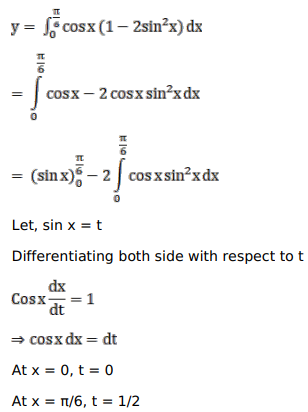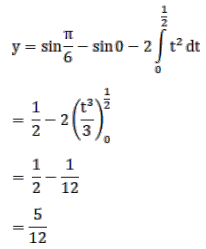# Mark against the correct answer in the following:`
Question:

Mark $(\sqrt{ })$ against the correct answer in the following:

$\int_{0}^{\pi / 6} \cos x \cos 2 x d x=?$

A. $\frac{1}{4}$

B. $\frac{5}{12}$

C. $\frac{1}{3}$

D. $\frac{7}{12}$

Solution: